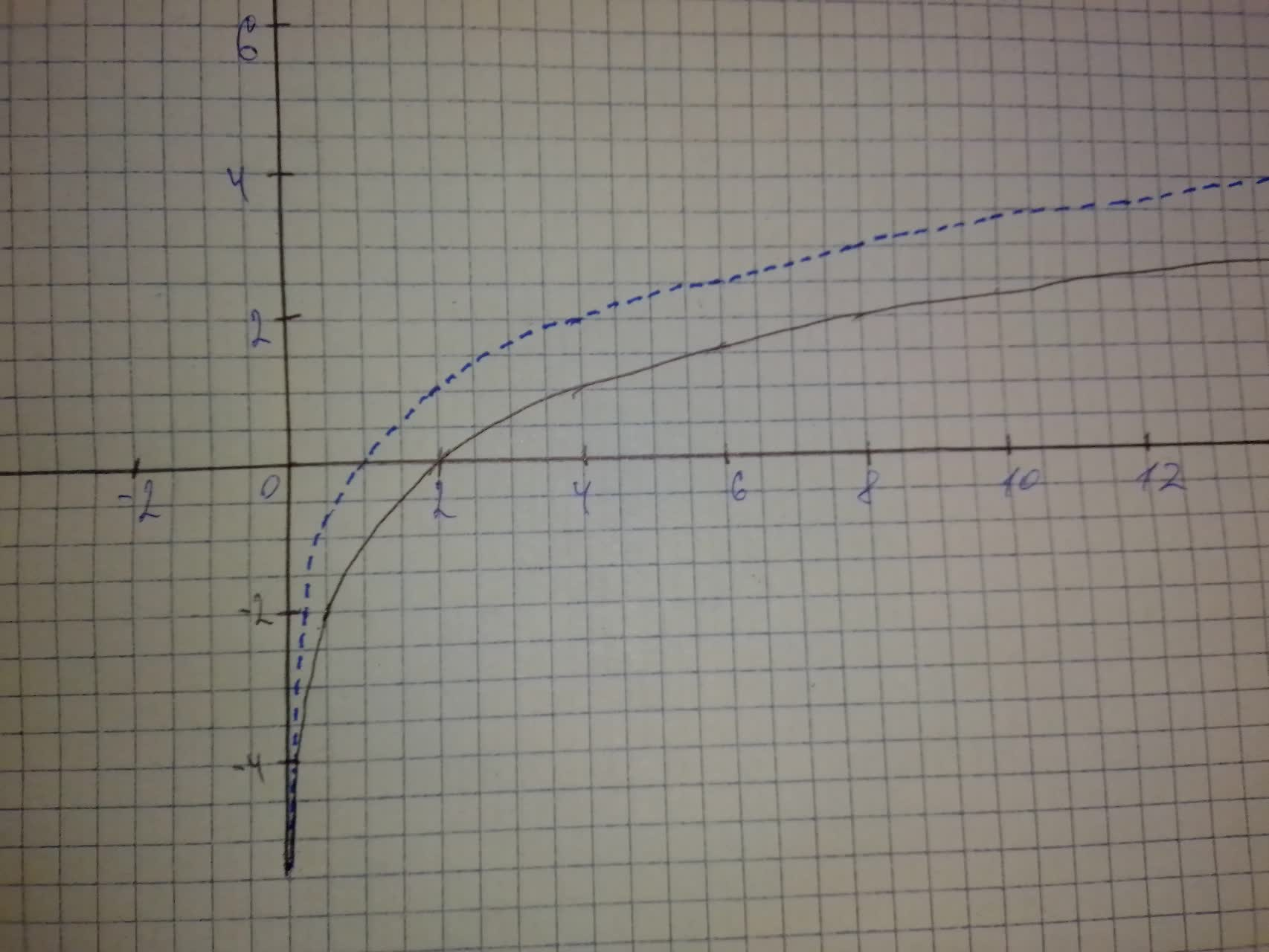Begin by graphing f(x)=\log_{2}x Then use transformations of this graph to graph the given function. What is the graph's x-intercept? What is the vertical asymptote?Annette Arroyo 2021-08-08 Answered
Begin by graphing $$\displaystyle{f{{\left({x}\right)}}}={{\log}_{{{2}}}{x}}$$.
Then use transformations of this graph to graph the given function. What is the graph's x-intercept? What is the vertical asymptote? Use the graphs to determine each function's domain and range.
$$\displaystyle{h}{\left({x}\right)}=-{1}+{{\log}_{{{2}}}{x}}$$

• Questions are typically answered in as fast as 30 minutes

Solve your problem for the price of one coffee

• Math expert for every subject
• Pay only if we can solve itAnonym
$$\displaystyle{h}{\left({x}\right)}=-{1}+{{\log}_{{{2}}}{\left({x}\right)}}$$ can be obtained from the graph of $$\displaystyle{{\log}_{{{2}}}{\left({x}\right)}}$$ by translating it along the x-axis by 1 unit in the downward direction
In the graph below:
The blue dotted curve represents the graph for $$\displaystyle{y}={{\log}_{{{2}}}{x}}$$
The black solid curve represents the graph for $$\displaystyle{y}=-{1}+{{\log}_{{{2}}}{x}}$$